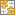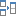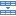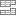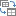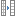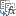# How to find top or bottom N values in Excel

With this tutorial, you will learn how to find top 3, 5, 10 or n values in a dataset and retrieve matching data.

Want to identify the highest or lowest N values in a column or row? That sounds like a pretty easy thing to do. Need to return not only the values themselves but their names too? Oh, that could be a challenge. There are one or more criteria to be met. Hmm, is that even possible?! Yep, all this can be done with Excel formulas and this tutorial shows how.

## Excel formula to find top 3, 5, 10, etc. values

To get the highest N values in a list, use the LARGE and ROWS functions together in this way:

LARGE(values, ROWS(A\$2:A2))

For example, to find top 3 values in B2:B12, you enter the below formula in the topmost cell of the range where you want the results to appear (D2), and then drag it through D4:

`=LARGE(\$B\$2:\$B\$12, ROWS(A\$2:A2))`

To get top 5 values, copy the same formula to 5 cells.

To find top 10 values in a column, copy the formula to 10 cells.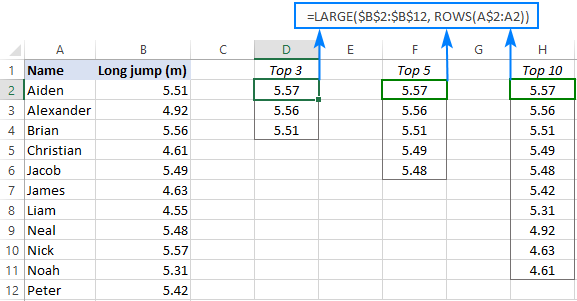How this formula works:

The LARGE function compares all the numeric values in a range supplied for the 1st argument (array) and returns the largest value based on the position specified in the 2nd argument (k).

The ROWS function with an expanding range reference like ROWS(A\$2:A2) generates the k values automatically. To create such a reference, we lock the row coordinate in the first cell (A\$2) and use a relative reference for the last cell (A2).

In the topmost cell where you enter the formula (D2 in this example), ROWS(A\$2:A2) generates 1 for k, telling LARGE to return the max value. When copied to the below cells, the range reference expands by 1 row causing the k argument to increment by 1. For example, in D3, the reference changes to A\$2:A3. The ROWS function counts the number of rows in A\$2:A3 and returns 2 for k, so the LARGE function outputs the 2nd largest value.

Please note that we used A\$2:A2 just for convenience because our data begins in row 2. You can use any column letter and any row number for the expanding range reference, say A\$1:A1 or C\$1:C1.

## Excel formula to get bottom 3, 5, 10, etc. values in Excel

To find the lowest N values in a list, the generic formula is:

SMALL(values, ROWS(A\$2:A2))

In this case, we use the SMALL function to extract the k-th smallest value and the ROWS function with an expanding range reference to generate the k number.

For example, to find bottom N values in the table below, use this formula:

`=SMALL(\$B\$2:\$B\$12, ROWS(A\$2:A2))`

Enter it in the topmost cell, and then drag down through as many cells as needed.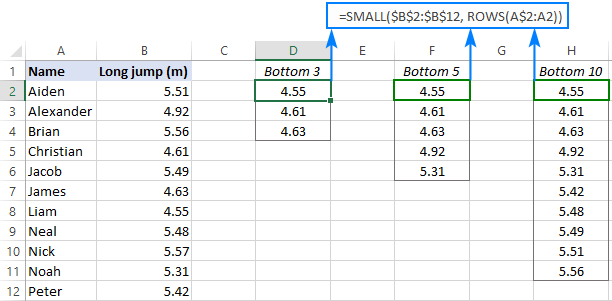## Excel formula to find top N values in a row

If your data is organized horizontally in rows, then you can use the following generic formulas to find the highest or lowest values:

Get top values in a row:

LARGE(values, COLUMNS(A\$2:A2))

Get bottom values in a row:

SMALL(values, COLUMNS(A\$2:A2))

The formulas' logic is the same as in the previous example with the difference that you use the COLUMNS function rather than ROWS to "feed" the k value to LARGE and SMALL.

Let's say your table lists the results of 5 rounds for each participant like shown in the image below. You aim to find top 3 values in each row.

To have it done, enter the following formula in the upper-right cell (B10 in our case), and then drag it down and to the right:

`=LARGE(\$B2:\$F2, COLUMNS(\$A1:A1))`

For the formula to copy correctly, we use a mixed reference for the array argument of LARGE that locks only the column coordinates (\$B2:\$F2).To find bottom 3 values in each row, you can use an analogous SMALL formula:

`=SMALL(\$B\$2:\$H\$2, COLUMNS(\$A2:A2))`

## How to get matches of largest N values

In situation when you want to retrieve data relating to top values, use the classic INDEX MATCH formula together with LARGE as the lookup value:

INDEX(return_array, MATCH(LARGE(lookup_array, k), lookup_array, 0))

Where:

• Return_array is a range from which to extract associated data (matches).
• Lookup_array is a range where to search for the largest values.
• K is position of the highest value to look for.

In the table below, you can find top 3 values by using the following approach.

To extract top 3 results, the formula in E3 is:

`=LARGE(\$B\$2:\$B\$12, D3)`

Because the ranks typed in separate cells, the ROWS function is not needed in this case - we simply reference the cell containing the k value (D3).

To retrieve the names, the formula in F3 is:

`=INDEX(\$A\$2:\$A\$12, MATCH(LARGE(\$B\$2:\$B\$12, D3), \$B\$2:\$B\$12, 0))`

Where A2:A12 are the names (return_array), B2:B12 are the results (lookup_array) and D3 is the position from largest (k).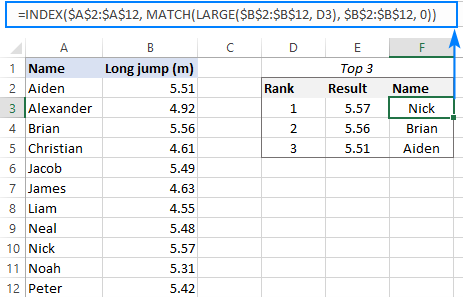In a similar manner, you can get matches of bottom N values. For this, just use the SMALL function instead of LARGE.

To get bottom 3 results, the formula in E3 is:

`=SMALL(\$B\$2:\$B\$12, D3)`

To pull the names, the formula in F3 is:

`=INDEX(\$A\$2:\$A\$12, MATCH(SMALL(\$B\$2:\$B\$12, D3), \$B\$2:\$B\$12, 0))`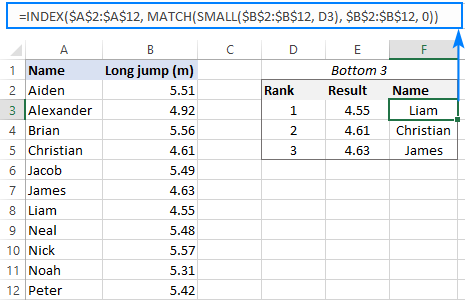How this formula works:

The LARGE function gets the k-th largest value and passes it to the lookup_value argument of MATCH.

For instance, in F3, we are looking for the 1st largest value, which is 5.57. So, after replacing the LARGE function with its output, the formula reduces to:

`=INDEX(\$A\$2:\$A\$12, MATCH(5.57, \$B\$2:\$B\$12, 0))`

The MATCH function determines the relative position of 5.57 in B2:B12, which is 9. This number goes to the row_num argument of INDEX, simplifying the formula further:

`=INDEX(\$A\$2:\$A\$10, 9)`

Finally, the INDEX function returns the value from the 9th row in the range A2:A12, which is "Nick".

#### XLOOKUP formula

Microsoft 365 subscribers can achieve the same results by using the new XLOOKUP function:

`=XLOOKUP(LARGE(\$B\$2:\$B\$12, D3), \$B\$2:\$B\$12, \$A\$2:\$A\$12)`

In this case, LARGE returns the k-th largest number directly to XLOOKUP as the lookup value.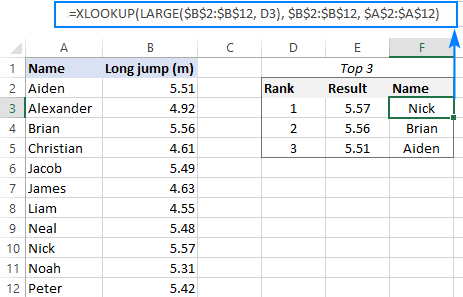Compared to the INDEX MATCH formula, this syntax is much simpler. However, please keep in mind that XLOOKUP is only available in Excel 365. In Excel 2019, Excel 2016 and earlier versions, this formula won't work.

## How to find top values with duplicates

The approach used in the previous example works fine for a dataset that has only unique numbers in the lookup column. Duplicates may lead to wrong results. For example, if the 1st and 2nd largest numbers happen to be the same, the LARGE function will return the same value for each, which is expected by design. But because MATCH returns the position of the first found match, the formula will output the first match twice like shown on the image below: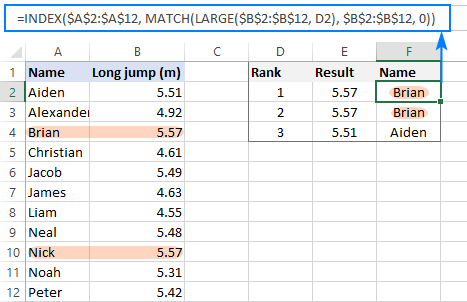To "break ties" and fix the problem, we need a more sophisticated MATCH function:

`=INDEX(\$A\$2:\$A\$12, MATCH(1, (\$B\$2:\$B\$12=LARGE(\$B\$2:\$B\$12, D2)) * (COUNTIF(F\$1:F1, \$A\$2:\$A\$12)=0), 0))`

In all versions except Excel 365, it only works as an array formula. So, please remember to press Ctrl + Shift + Enter to complete the formula correctly.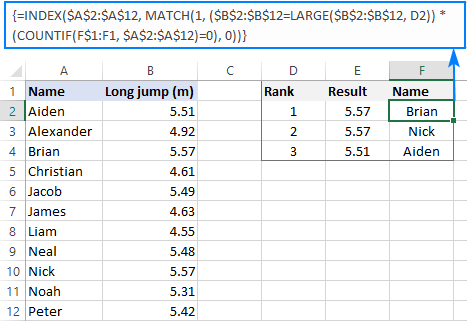How this formula works:

Here, the MATCH function is configured to search for the number 1, which is the lookup value. The lookup array is constructed using the following logic:

Firstly, all the numbers are compared against the value returned by LARGE:

`\$B\$2:\$B\$12=LARGE(\$B\$2:\$B\$12, D2)`

Secondly, the COUNTIF function with an expanding range reference checks if a given item is already in the top list. Please pay attention that the expanding range begins on the above row (F1) to avoid a circular reference.

`COUNTIF(F\$1:F1, \$A\$2:\$A\$12)=0`

The result of the above operations are two arrays of TRUE and FALSE values, which are multiplied by each other. For example, in F3, we have the following arrays:

`{FALSE;FALSE;TRUE;FALSE;FALSE;FALSE;FALSE;FALSE;TRUE;FALSE;FALSE} * {TRUE;TRUE;FALSE;TRUE;TRUE;TRUE;TRUE;TRUE;TRUE;TRUE;TRUE}`

In the first array, there are two TRUE values that correspond to 5.57 (rank 1 and 2) - items 3 and 9. But in the second array, item 3 is FALSE because this name (Brian) is already in the list. The multiplication operation changes the logical values TRUE and FALSE into 1 and 0, respectively. And because multiplying by zero always gives zero, only item 9 "survives":

`{0;0;0;0;0;0;0;0;1;0;0}`

The MATCH function searches for "1" in this array, and returns its relative position (9), which is Nick.

Note. This solution implies that the return column (Name in our case) contains only unique values.

## How to find top values in Excel with criteria

To get top values that match certain condition, express your criteria with the help of the IF function and nest them inside the formulas discussed in the previous sections.

As an example, let's find top 3 results in a given group. To have it done, we input the target group in F1 and type the ranks 1 to 3 in E5:E7 (please see the image below).

To extract top 3 results, enter the below formula in F5 and drag it though F7:

`=LARGE(IF(\$B\$2:\$B\$12=\$F\$1, \$C\$2:\$C\$12), E5)`

To ensure that the LARGE function is only processing the results within the target group, we build an IF statement that compares a list of groups against F1.

To get the names, copy this formula in G5 through G7:

`=INDEX(\$A\$2:\$A\$12, MATCH(LARGE(IF(\$B\$2:\$B\$12=\$F\$1, \$C\$2:\$C\$12), E5), IF(\$B\$2:\$B\$12=\$F\$1, \$C\$2:\$C\$12), 0))`

Here, we use the already familial INDEX MATCH LARGE combination but extend it with two logical tests:

LARGE(IF(\$B\$2:\$B\$12=\$F\$1, \$C\$2:\$C\$12), E5) - IF checks if a group matches the target one in F1. The matches get into the array from which the LARGE function picks the highest value based on the rank in E5. The result becomes the lookup value for MATCH.

IF(\$B\$2:\$B\$12=\$F\$1, \$C\$2:\$C\$12) - IF filters out irrelevant groups again, so only the results that belong to the target group make it into the lookup array, where MATCH searches for the lookup value.

These are array formulas that should be entered by pressing Ctrl + Shift + Enter simultaneously. Due to the ability of Excel 365 to handle arrays natively, it's sufficient to press the Enter key in this version.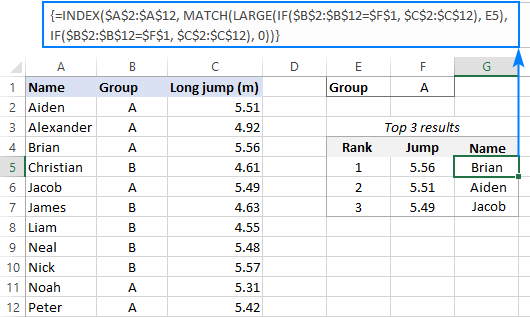In Excel 365, you can perform the same logical tests inside XLOOKUP and get the identical results:

`=XLOOKUP(LARGE(IF(\$B\$2:\$B\$12=\$F\$1, \$C\$2:\$C\$12), E5), IF(\$B\$2:\$B\$12=\$F\$1, \$C\$2:\$C\$12), \$A\$2:\$A\$12)`

Tip. To find bottom values with criteria, simply replace LARGE with SMALL in the above formulas.

## How to FILTER top or bottom values in Excel

In Excel 365, there is a simpler way to find largest N values by using new dynamic array functions such as SORT and FILTER.

SORT(FILTER(data, numbers>=LARGE(numbers, n)), sort_index, -1)

Where:

• Data is the source table excluding column headers.
• Numbers are the numeric values to rank.
• N is the number of top entries to extract.
• Sort_index the number of the column to sort by.

For example, to filter top 3 records in our set of data, the formula goes as follows:

`=SORT(FILTER(A2:B12, B2:B12>=LARGE(B2:B12, 3)), 2, -1)`

In this case, we set n to 3 because we're extracting top 3 results and sort_index to 2 since the numbers are in the second column.

The beauty of this formula is that you only need to enter it in one cell, and Excel automatically spills the results into as many cells as needed (this concept is called a spill range).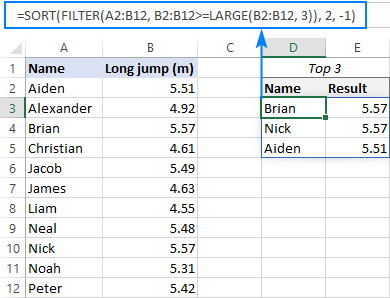To extract the lowest 3 results, the formula is:

`=SORT(FILTER(A2:B12, B2:B12<=SMALL(B2:B12, 3)), 2, 1)`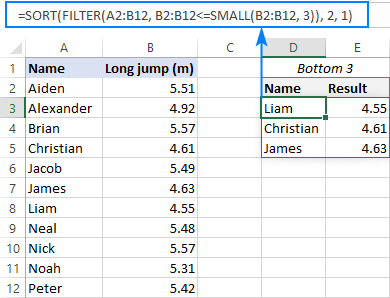How these formulas work:

Here, we use the FILTER function to filter the source data based on the criteria included in the 2nd argument.

To get top values, we construct the logical expression that checks if a given number is greater than or equal to the 3rd highest number in the list: B2:B12>=LARGE(B2:B12, 3).

To get bottom values, we check if a number is less than or equal to the 3rd lowest number: B2:B12<=SMALL(B2:B12, 3).

The result of the logical test is an array of TRUE and FALSE values, which is used for filtering - only the entries corresponding to TRUE make it into the final array:

`=SORT({"Aiden",5.51;"Brian",5.57;"Nick",5.57}, 2, -1)`

As you can see above, the FILTER function outputs the items in the same order they appear in the source range. To sort the results, we place the FILTER formula inside the SORT function and set the sort_order argument to either -1 (descending) or 1 (ascending).

That's how to find top values in Excel. I thank you for reading and look forward to seeing you on our blog next week!

Excel formulas to find top or bottom values (.xlsx file)

## You may also be interested in:

### 2 responses to "How to find top or bottom N values in Excel"

1.Yulia says:

Hello Svetlana,

Thank you very much for the posts! I have just started exploring the website looking for some personal tips for work with excel, and I must say that the format of the posts is very nice! Especially, thank you for taking time and posting screenshots with the clear explanations!

•Svetlana Cheusheva (Ablebits.com Team) says:

Thank you for your positive feedback, Yulia! It's the best incentive for us to keep up and improve :)

Ultimate Suite for Microsoft Excel